## Thursday, June 25, 2015

### Customizing your contMap style visualization using phytools: User questions & answers

A phytools user recently contacted me with the following questions about the phytools function `contMap` for mapping the evolution of continuous trait on the tree using a color gradient:

a) Does the “length” value in the legend specify how many millions of years the legend bar represents?
b) If so, is it possible to suppress the length call and and have an additional time scale across the whole tree instead?
c) Is there any way to name the trait value?
d) Is there a simple way to change the colour, on which one maps the trait?

Since these questions probably come up for other users as well, I thought I'd try to address them one by one here.

First, let's review the function `contMap`. Here is a quick demo illustrating it's use, which I will explain further below:

``````library(phytools)
## simulate a tree
tree<-pbtree(n=26,tip.label=LETTERS,scale=100)
plotTree(tree)
``````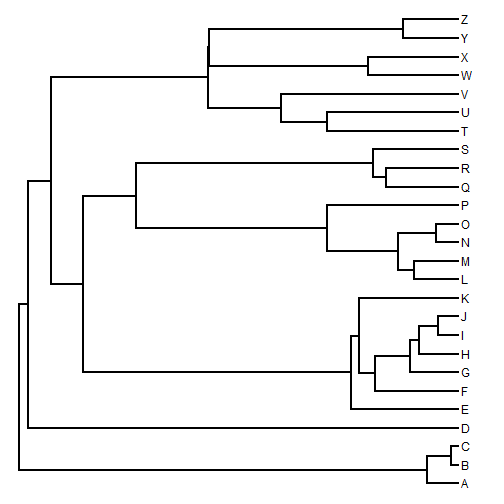``````## simulate some character data
x<-fastBM(tree,sig2=0.1)
x
``````
``````##          A          B          C          D          E          F
## -5.2978022 -6.1858831 -5.7305370 -2.0332898 -4.2643267 -0.8574406
##          G          H          I          J          K          L
## -2.2867929 -1.1968481 -0.8374673 -0.6996979 -3.6258800  2.2016812
##          M          N          O          P          Q          R
##  2.3865797  3.0411627  2.9741112  0.5108229  0.8685003  1.6050838
##          S          T          U          V          W          X
##  3.1910221 -0.5765872  1.0726064 -1.2610571 -0.5643860  0.4667727
##          Y          Z
##  2.5718508  2.5810971
``````
``````## generate an object of class "contMap"
obj<-contMap(tree,x,plot=FALSE)
obj
``````
``````## Object of class "contMap" containing:
##
## (1) A phylogenetic tree with 26 tips and 25 internal nodes.
##
## (2) A mapped continuous trait on the range (-6.185883, 3.191022).
``````
``````## plot it usin default settings:
plot(obj)
``````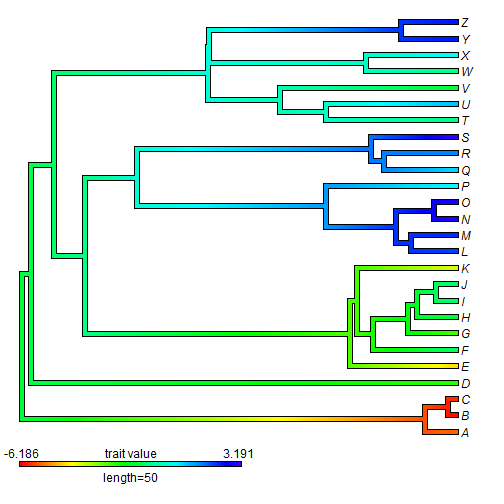The first question was: “Does the "length” value in the legend specify how many millions of years the legend bar represents?“

The answer is "yes” - if our branch lengths are in millions of years. Otherwise it will be in whatever units we have for the edge lengths of the tree. We can verify this by replotting our object of class `"contMap"`, while leaving enough space for a horizontal axis which we can then add to the plot for comparison. We can even change the length of the plotted legend as follows:

``````plot(obj,mar=c(5.1,0.2,0.2,0.2),legend=40)
axis(1)
title(xlab="time from the root")
``````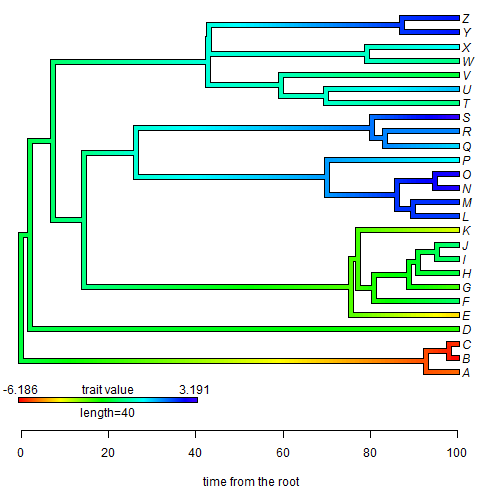The second question was then: “If so, is it possible to suppress the length call and and have an additional time scale across the whole tree instead?”

Well, I've just (above) demonstrated how to include a labeled x-axis in the plot. What I think the user really wants to do is suppress the text below the `contMap` legend. This is possible, but a little trickier. Here's how we can do it:

``````## first plot our tree sans legend
plot(obj,legend=FALSE,ylim=c(1-0.09*(Ntip(obj\$tree)-1),Ntip(obj\$tree)),
mar=c(5.1,0.4,0.4,0.4))
## now add our legend, but without the text underneath
lims=obj\$lims,digits=3,prompt=FALSE,x=0,
y=1-0.08*(Ntip(obj\$tree)-1),lwd=4,fsize=1,subtitle="")
axis(1)
title(xlab="time from the root")
``````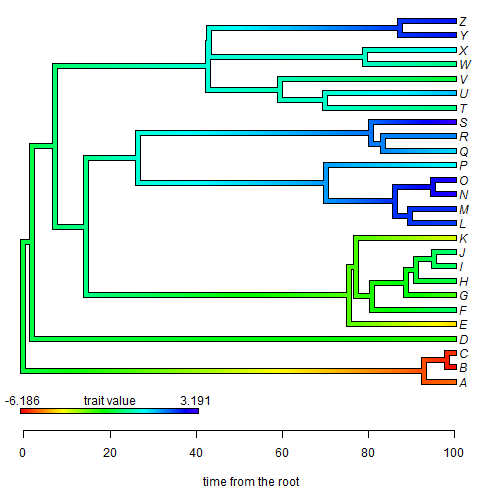The next question was: “Is there any way to name the trait value?”

Well - from the example above, it should be obvious that this is possible. Let's combine them together - but, let's call our trait (for example) log(body size):

``````plot(obj,legend=FALSE,ylim=c(1-0.09*(Ntip(obj\$tree)-1),Ntip(obj\$tree)),
mar=c(5.1,0.4,0.4,0.4))
lims=obj\$lims,digits=3,prompt=FALSE,x=0,
y=1-0.08*(Ntip(obj\$tree)-1),lwd=4,fsize=1,subtitle="")
axis(1)
title(xlab="time from the root")
``````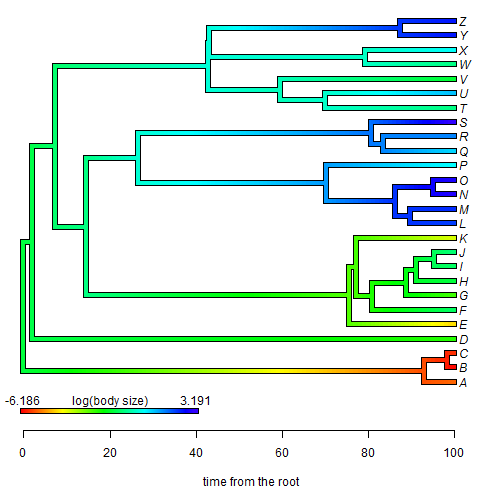Finally, the user asked: “Is there a simple way to change the colour, on which one maps the trait?” This one is actually pretty straightforward as there is a custom function in phytools, `setMap` for this. Let's change our rainbow color map to a blue-purple-red map:

``````obj<-setMap(obj,colors=c("blue","purple","red"))
## plot under default conditions
plot(obj)
``````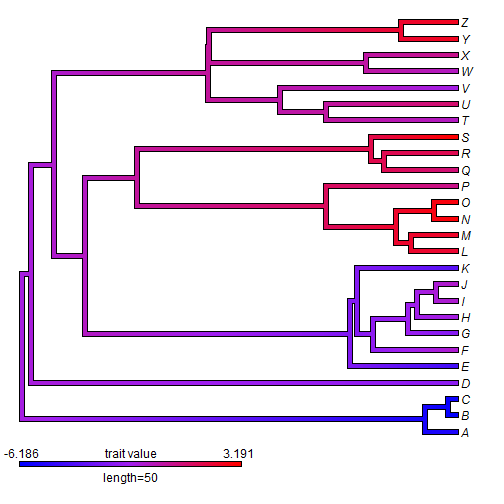## Wednesday, June 24, 2015

### New S3 print/plot methods & new functionality for phytools ltt

I just updated the phytools function `ltt`, which computes and plots a lineage-through-time plot, even for trees that contain lineages terminating before the present day (i.e., extinct lineages).

The main changes in this version is that I created new object classes, `"ltt"` (for a single lineage-through-time plot) or `"multiLtt"` (for two or more trees supplied as an object of class `"multiPhylo"`, and then created S3 `print` and `plot` methods for these object classes.

From a practical standpoint, the main functionality added is that the arguments of `plot.default` can now be used to customize the LTT visualization. Here is a quick demo of the new methods and the new functionality of `ltt`:

``````library(phytools)
``````
``````## Loading required package: ape
``````
``````packageVersion("phytools")
``````
``````##  '0.4.58'
``````
``````## basic ltt plotting
tree<-pbtree(n=26,tip.label=LETTERS,scale=10)
obj<-ltt(tree,plot=FALSE)
## S3 print method
obj
``````
``````## Object of class "ltt" containing:
##
## (1) A phylogenetic tree with 26 tips and 25 internal nodes.
##
## (2) Vectors containing the number of lineages (ltt) and branching times (times) on the tree.
##
## (3) A value for Pybus & Harvey's "gamma" statistic of -1.1127, p-value = 0.2658.
``````
``````## S3 plot method
plot(obj,lwd=2,log.lineages=FALSE,log="y",
main="lineage through time plot")
``````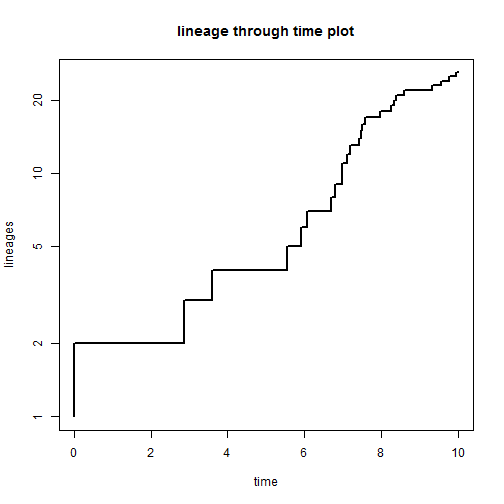``````## tree with extinction
tree<-pbtree(n=100,b=1,d=0.4,t=4)
``````
``````## simulating with both taxa-stop (n) and time-stop (t) is
## performed via rejection sampling & may be slow
##
##   2806 trees rejected before finding a tree
``````
``````obj<-ltt(tree,plot=FALSE,gamma=FALSE)
obj
``````
``````## Object of class "ltt" containing:
##
## (1) A phylogenetic tree with 143 tips and 142 internal nodes.
##
## (2) Vectors containing the number of lineages (ltt) and branching times (times) on the tree.
``````
``````plot(obj,log.lineages=FALSE,log="y",
ylab="number of lineages (log-scale)")
``````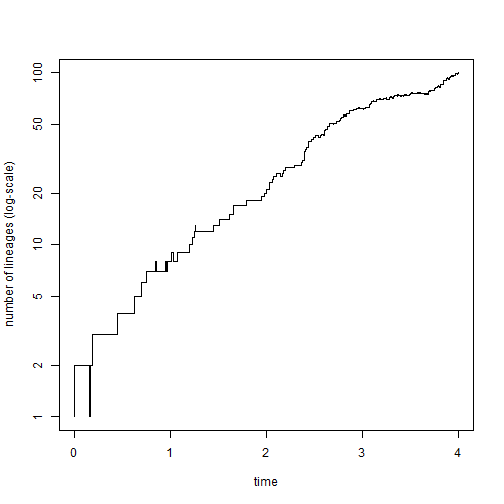``````## multiple trees
trees<-pbtree(n=40,scale=1,nsim=200)
obj<-ltt(trees)
``````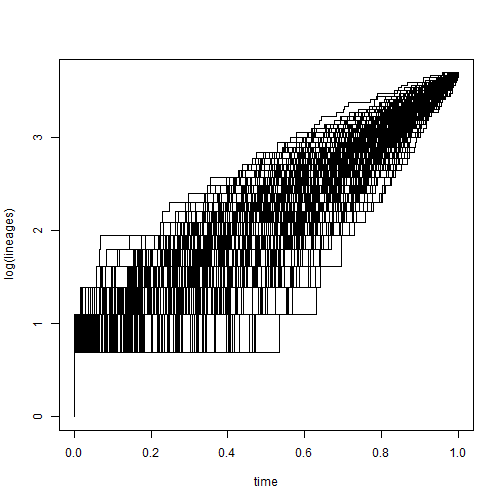``````obj
``````
``````## 200 objects of class "ltt" in a list
``````
``````## distribution of gamma
gamma<-sapply(obj,function(x) x\$gamma)
hist(gamma,main="distribution of gamma from 200 simulations")
``````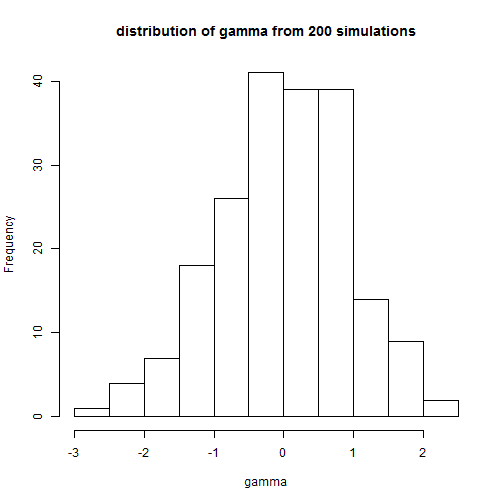``````plot(obj,log.lineages=FALSE,
main="lineage through time plots for 200 trees",
col=rgb(0,0,1,0.1)) ## plot lines with transparency
``````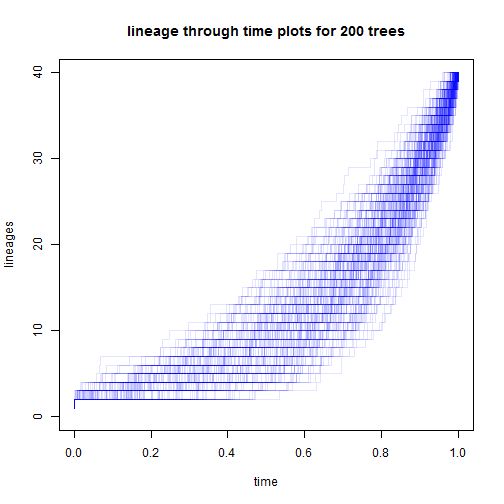The main technical challenge that I foresee is that I use the function `do.call` internally to execute `plot.default` and `lines`, depending on whether or not the lineage-through- time plot is to be added to the current graphical device. However, `plot` and `lines` take different arguments, so I can see that some users may be able to generate errors by supplying arguments that can be handled by `plot` but not by lines when the object being plotted is of class `"multiLtt"`! Please report this if you encounter it.

The code for this version of the function & associated methods is posted here, and the latest phytools package version build can be downloaded from the phytools page.

OK, that's it for now!